Next: Processing the sensitivity Up: Sensitivity Analysis Previous: Sensitivity Analysis   Contents

### Preprocessing the sensitivity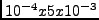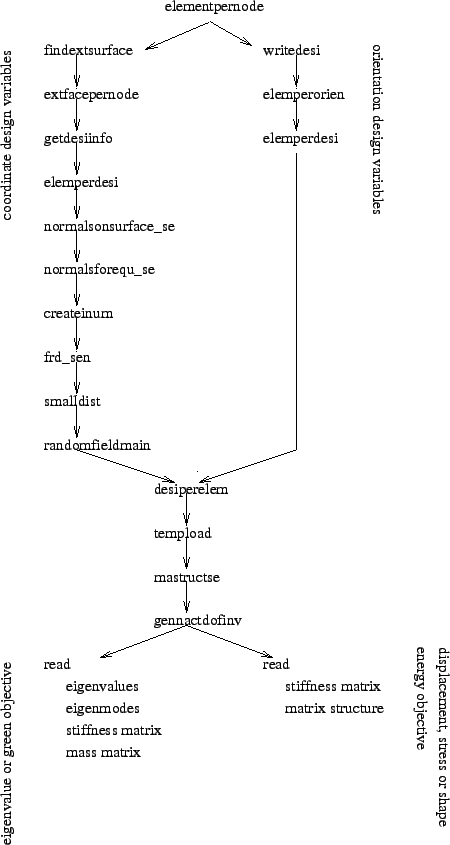The Structure of the preprocessing part is shown in Figure 174. At first all elements belonging to one and the same node are determined and stored in the data structure shown in Figure 175. Then, the program flow is split according to whether the design variables are the coordinates or the material orientations.

For coordinate design variables the following steps are performed:

• The external faces of the structure are determined and stored in the data format explained in Figure 164 as well as in the data format shown in Figure 176 (findextsurface.f)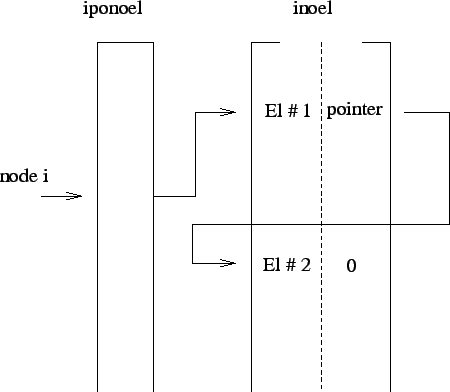• All external faces to which a given node belongs are stored in fields iponoelfa and inoelfa according to the data structure shown in Figure 177 (extfacepernode.f)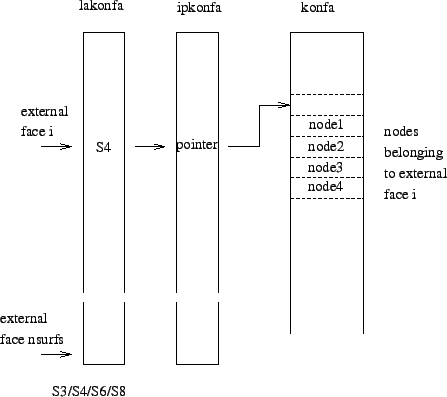• The design variables (i.e. nodes) are stored in ascending order in field nodedesi(*). The total number of design variables is ndesi (getdesiinfo.f)

• All elements belonging to one and the same design variable are stored in fields istartdesi and ialdesi according to the structure in Figure 178 (elemperdesi.f)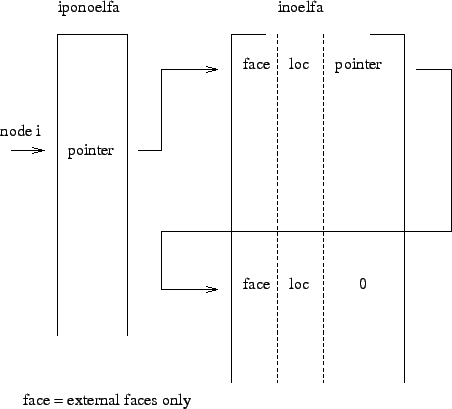• The calculation of the normals to the external surfaces. At the design variables, this is the direction in which the nodes are moved and for which the sensitivity is calculated. In each node there can be only one normal. This is the mean of the normals on all external faces to which the node belongs. If the EDGE PRESERVATION=YES parameter is activated on the *FILTER card only external surfaces internal'' to the domain'' of design variables are taken into account for the calculation of the normal. An external surface is internal'' to the domain'' of more than half of its nodes are design variables (normalsonsurface_se.f).

• The calculation of the normals to the external surfaces. Although it seems to be the same task as in the previous item, it is not. The normals calculated here are needed for the mesh modifications in an optimization procedure. Usually the performance of a sensitivity study is not a goal itself, rather it is part of an optimization loop during which the sensitivities, which are nothing else than the derivative of the objective w.r.t. the design variables, are recalculated in each iteration and used in optimization strategies such as steepest descent or conjugate gradient. At the end of each iteration the design variables are moved a small amount in the normal direction (calculated in the previous item), all other nodes are not moved in normal direction. This deforms the mesh and may lead to bad elements. Hence the mesh has to be improved, e.g. with a Laplace operator. However, the external surface of the structure should not be changed during this operation. This latter requirement can be taken care of by defining multiple point constraints based on the local normal(s). Indeed, now more than one normal may be needed, e.g. at sharp corners which need be preserved. Therefore, the way the normals are determined here (normalsforequ_se.f) is different from the way this is done in normalsonsurface_se.f. The equations are stored in file jobname.equ for further use in a CalculiX input deck.

• Determination of the active nodes, i.e. the nodes belonging to elements (createinum.f) and storage of the normals determined in normalsonsurface_se.f in frd-format in jobname.frd (frd_sen.c).

• Determination of the smallest distance between two nodes belonging to one and the same element. Based on this distance a measure is derived (actually this distance/10000.) which is used to calculate the sensitivities on element-to-element basis with finite differences (smalldist.f).

• randomfieldmain.f

For orientation design variables the following steps are performed:

• Storage of the orientation design variables in the jobname.dat file. Each local orientation leads to exacty three design variables, which are the components of the rotation vector describing the orientation (writedesi.f).

• Determining all elements corresponding to a given orientation. They are stored in fields ipoorel(*) and iorel(2,*) in exactly the same way as fields iponoel(*) and inoel(2,*) were used to store all elements to which a given node belongs, cf. Figure 175 (elemperorien.f).

• All elements belonging to one and the same design variable are stored in fields istartdesi and ialdesi according to the structure in Figure 178 (elemperdesi.f). This is analogous to the case in which the coordinates are the design variables.

The next four routines are common to coordinate design variables as well as orientation design variables:

• First the design variables per element are determined and stored in fields istartelem(*) and ialelem(*) in exactly the same way as fields istartdesi(*) and ialdesi(*) were used to store the elements per design variable according to Figure 178 (desiperelem.f).

• The actual external load is determined (tempload.f)

• The matrix structure of the sensitivity matrix df is determined and stored using the variables irows(*) and jqs(*). The sensitivity matrix is used to store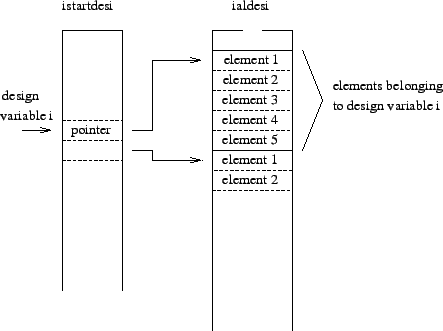,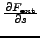,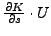or a combination of these. The dimensions are neq x ns, where neq is the number of independent degrees of freedom and ns is the number of design variables. This matrix is very sparse, since only the degrees of freedom belonging to the nodes to which the design variable belongs will be nonzero. The nonzero's are stored column by column according to ascending row numbers for each column. Field irows contains the corresponding row numbers (size = total number of nonzero's), field jqs(i) contains the location of the first entry in irows beloning to column i.

• Each degree of freedom in field df corresponds to a specific direction in a specific node. In gennactdofinv.f field nactdofinv(i) is determined yielding the direction and node for a degree of freedom i in the form (node-1)*mt+direction, where mt is the maximum number of directions (=mi(2), cf. List of variables and their meaning) + 1.

At this point the preprocessing part is split according to whether the objectives are the eigenvalues or Green functions, in which case the eigenvalues, eigenmodes, stiffness matrix and mass matrix are read from file (generate in a previous *FREQUENCY or *GREEN step), or whether the objective is the mass, the stress or the shape energy, in which case the stiffness matrix and the matrix structure are read from file (generated in a previous *STATIC step).Next: Processing the sensitivity Up: Sensitivity Analysis Previous: Sensitivity Analysis   Contents
guido dhondt 2018-12-15